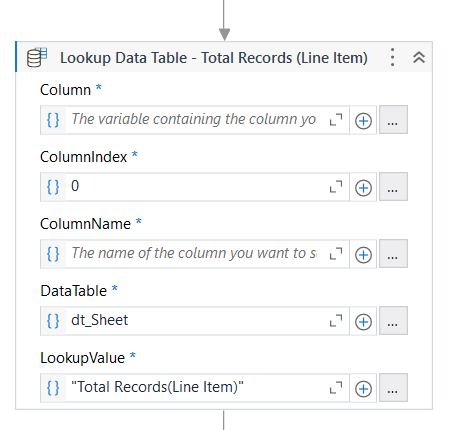# LINQ to lookup string and get value after ":"

Based on the pictures, I need 6 values. For the last two values, it needs to be referenced by “Partner System Data Error” since there’s another same thing exists but were referenced by “Error 12”

I tried using Lookup Data Table activity for all 6 values, but it returned nothing.Here’s attached the input file.
Input.xlsx (10.8 KB)

@arina

Please use filter datatable to get the values and then use split activity to split the data with colon(and get the data

in filter datatble you can use contains filter to filter on the required lines…

cheers

Hi @arina ,

Maybe an alternate would be to use regex methods on the whole input to capture the required values.

Check the below Steps :

1. Read the Excel Sheet using `Read Range` Activity, get the Output as datatable value say `DT`.

2. Next, use `Output Datatable` Activity and get the Output String, say `strDT`, to convert the datatable data to a String data. We can now apply String/Regex methods on this output.

3. Applying Regex Expressions on the Data to get the Required value :

Expressions to capture each required Value :

``````TotalRecords = Regex.Match(strDT,"(?<=Total Records\(Line Item\)\s+:).*",RegexOptions.IgnoreCase).Value.Trim

TotalAmtLineItem = Regex.Match(strDT,"(?<=Total Amount at Line Item\s+:).*",RegexOptions.IgnoreCase).Value.Trim

TotalRecordsProcessed = Regex.Match(strDT,"(?<=Total Records\(Line Item\)Processed\s+:).*",RegexOptions.IgnoreCase).Value.Trim

TotalAmtLineItemProcessed = Regex.Match(strDT,"(?<=Total Amount\s+Line Item Processed\s+:).*",RegexOptions.IgnoreCase).Value.Trim

TotalRecordsNotProcessed = Regex.Match(strDT,"(?<=Total Records\(Line Item\)  Not Processed\s+:).*",RegexOptions.IgnoreCase).Value.Trim

TotalAmtLineItemNotProcessed = Regex.Match(strDT,"(?<=Total Amount\s+Line Item Not Processed\s+:).*",RegexOptions.IgnoreCase).Value.Trim
``````

Let us know if you are not able to get the output.Is this normal to have the highlighted one? Only the first four have these.